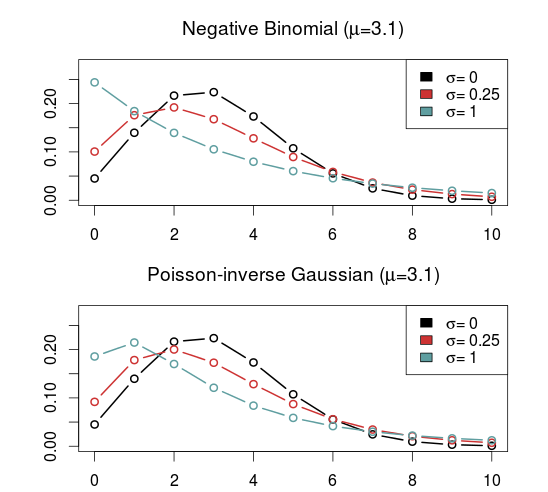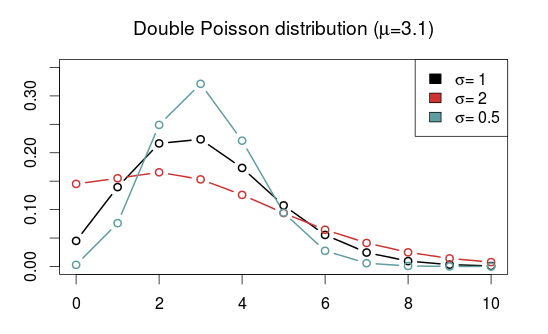# Some alternatives to the Poisson distribution

One important characteristic of the Poisson distribution is that both its expectation and the variance equals parameter $$\lambda$$. A consequence of this is that when we use the Poisson distribution, for example in a Poisson regression, we have to assume that the variance equals the expected value.

The equality assumption may of course not hold in practice and there are two ways in which this assumption can be wrong. Either the variance is less than the expectation or it is greater than the expectation. This is called under- and overdispersion, respectively. When the equality assumption holds, it is called equidispersion.

There are two main consequences if the assumption does not hold: The first is that standard errors of the parameter estimates, which are based on the Poisson, are wrong. This could lead to wrong conclusions when doing inference. The other consequence happens when you use the Poisson to make predictions, for example how many goals a football team will score. The probabilities assigned to each number of goals to be scored will be inaccurate.

(Under- and overdispersion should not be confused with heteroscedasticity in ordinary linear regression. Poisson regression models are naturally heteroscedastic because of the variance-expectation equality. Dispersion refers to what relationship there is between the variance and the expected value, in other words what form the heteroscedasticity takes.)

When it comes to modeling and predicting football results using the Poisson, a good thing would be if the data were actually underdispersed. That would mean that the probabilities for the predicted number of goals scored would be higher around the expectation, and it would be possible to make more precise predictions. The increase in precision would be greatest for the best teams. Even if the data were really overdispersed, we would still get probabilities that more accurately reflect the observed number of goals, although the predictions would be less precise.

This is the reason why I have looked into alternatives to the Poisson model that are suitable to model count data and that are capable of being over- and underdispersed. Except for the negative binomial model there seems to have been little focus on more flexible Poisson-like models in the literature, although there are a handful of papers from the last 15 years with some applied examples.

I should already mention the gamlss package, which is an extremely useful package that can fit a large number of regression type models in R. I like to think of it as the glm function on steroids. It can be used to create regression models for a large number of distributions (50+) and using different forms of dependent variables (for example random effects and splines) and doing regression on distribution parameters other than the usual expectation parameters.

The models that I have considered usually have two parameters. The two parameters are often not easy to interpret, but the distributions can be re-parameterized (which is done in the gamlss package) so that the parameters describe the location (denoted $$\mu$$, often the same as the expectation) and shape (denoted $$\sigma$$, often a dispersion parameter that modifies the association between the expectation and variance). Another typical property is that they equal the Poisson for certain values of the shape parameter.

As I have already mentioned, the kind of model that is most often put forward as an alternative to the Poisson is the Negative binomial distribution (NBI). The advantages of the negative binomial are that is well studied and good software packages exists for using it. The shape parameter $$\sigma > 0$$ determines the overdispersion (relative to the Poisson) so that the closer it is to 0, the more it resembles the Poisson. This is a disadvantage as it can not be used to model underdispersion (or equidispersion, although in practice it can come arbitrarily close to it). Another similar, but less studied, model is the Poisson-inverse Gaussian (PIG). It too has a parameter $$\sigma > 0$$ that determines the overdispersion.A large class of distributions, called Weighted Poisson distributions, is capable of being both over- and underdispersed. (The terms Weighted in the name comes from a technique used to derive the distribution formulas, not that the data is weighted) A paper describing this class can be found here. The general form of the probability distribution is

$$P(x;\theta,\alpha)=\frac{e^{\mu x+\theta t(x)}}{x!C(\theta,\alpha)}$$

where $$t(x)$$ is one of a large number of possible functions, and $$C(\theta,\alpha)$$ is a normalizing constant which makes sure all probabilities in the distribution sum to 1. Note that I have denoted the two parameters using $$\theta$$ and $$\alpha$$ and not $$\mu$$ and $$\sigma$$ to indicate that these are not necessarily location and shape parameters. I think this and interesting class of distributions that I want to look more into, but since they are not generally implemented in any R package that I know of I will not consider them further now.

Another model that is capable of being over- and underdispersed is the Conway–Maxwell–Poisson distribution (COM), which incidentally is a special case of the class of Weighted Poisson distributions mentioned above (see this paper). The Poisson distribution is a special case of the COM when $$\sigma = 1$$, and is underdispersed when $$\sigma > 1$$ and overdispersed when $$\sigma$$ is between 0 and 1. One drawback with the COM model is that the expected value depends on both parameters $$\mu$$ and $$\sigma$$, although it is dominated by $$\mu$$. This makes the interpretation a bit difficult, but it may not be a problem when making predictions.

Unfortunately, the COM model is not supported by the gamlss package, but there are some other R packages that implements it. I have tried a few of them and the only one that I got to work is CompGLM, which for some reason does not use the location ($$\mu$$) and shape ($$\sigma$$) parameterization.The Double Poisson (DP) is another interesting distribution which also equals the Poisson distribution when $$\sigma = 1$$, but is overdispersed when $$\sigma > 1$$ and underdispersed when $$\sigma$$ is between 0 and 1. The expectation does not depend on the shape parameter $$\sigma$$, and it is approximately equal to the location parameter $$\mu$$. Another interesting thing about the Double Poisson is that it is belongs to a larger group of distributions called double exponential families which also lets you derive a binomial-like distribution with an extra dispersion parameter which can be useful in a logistic regression setting (see this paper, or this preprint).In a follow up post I will try to use these distributions in regression models similar to the independent Poisson model.

## 14 thoughts on “Some alternatives to the Poisson distribution”

1.javier on said:

Hi Jonas,

We wrote a short piece about it some time ago:
http://blog.kickdex.com/post/52627445903/what-are-the-germans-doing-differently

One of the sources on the topic would be this paper:
http://www.sciencedirect.com/science/article/pii/S0378437102010300

•opisthokonta on said:

I haven’t thought of that before. I guess my main skepticism against using GEV distributions are that (as far as I know) they are continuous and football goals are discrete/counting variables. But I guess you could always try to do some discretization.

Also, I should probably have mentioned that this blog about comparing football and rugby scores was my main inspiration for looking for alternatives and introducing me for the gamlss package.

2.alfonso on said:

You listened a couple of poisson distributions to handle over/underdispersion. What makes you think that this is appropriate for modelling soccer goals?

I did fit the english leagues with R glm.nb and theta -> infinity, with some adjustedment of the maxiter in glm.control and set an initial value. With theta -> infinity the NB distribution becomes poisson.

•opisthokonta on said:

I wasn’t really sure it was appropriate, but I thought it might be worth looking into. My hunch was that when you know who plays who it might be that you actually have enough information that you could make better predictions when you don’t have to make the equidispersion assumption.

The NB distribution can only be overdispersed, so when you estimate theta to be infinity, i.e. you have a Poisson, you have not ruled out that it could be underdispersed. I am currently working on a blog post where I fit a couple of these models, and it looks like the underdispersed models often performs better.

•alfonso on said:

The generalized poisson distribution GPD can handle both and is quite common.

The derivation of the log is easy to do. However the inequality condition requires an advance solver or some additional math of the lagrange multiplicator.

•alfonso on said:

I tried it and the home goals got lambda = -0.018 for the english premiere league. Note, that lambda and theta is switched in the GPD. The lambda for the away goals got not distinct from 0.
The m parameter works like a “truncation”. I set m to ceil(max(goals)). With min(theta) = 0.5 minimum expected goal rates (old lambda in poisson distr.) you could use a fixed lower bound, ie. -min(theta) /m = 0.5/7 = -0.07.
Hope that helps

•opisthokonta on said:

That one looks interesting, strange I haven’t seen it before. The definition given in that book looks a bit complicated, with the truncation parameter m and the complicated bounds on the lambda parameter, but this paper that I found gives the formulation without the truncation and bounds. I will definitely look more into that one. Thanks!

•alfonso on said:

The version in the paper only handles the positive case, ie. overdispersion. Appart from that both are the same. The parameter is easy to come by. Just fit a regular poisson model. Take the min(lambda) and then divide my max(goals)+1. This is your lower bound.
Don’t worry your parameter fit never will hit that border.

Here’s a fantastic free solver:
http://users.iems.northwestern.edu/~nocedal/lbfgsb.html
With an interface to python:
http://docs.scipy.org/doc/scipy/reference/generated/scipy.optimize.fmin_l_bfgs_b.html

The only thing left to learn is to take the derivative of the log-function. Its easy to start with the poisson. Once getting used to it, you can fit any probability function of choice.

•opisthokonta on said:

Thanks for the clarification. The L-BFGS-B optimizer is also available from the optim function in R.

3.Antonio José Sáez-Castillo on said:

We have specifically employed the Generalized Waring Regression Model (GWRM) to fit the number of goals scored by footballers (http://www.sciencedirect.com/science/article/pii/S016794730900125X): you’ll find its R implementation at https://cran.r-project.org/web/packages/GWRM/GWRM.pdf
Moreover, we have explored another extension of the Poisson regression model which cope with under- and over-dispersion using hyper-Poisson distributions (http://www.sciencedirect.com/science/article/pii/S0167947312004434). Unfortunately, we are far from being able to offer an R package yet, although single R functions to fit the model to data are located at http://www4.ujaen.es/~ajsaez/hp.fit.r (see examples at http://www4.ujaen.es/~ajsaez/hp.examples.R)

•opisthokonta on said:

I remember I read your paper on the Waring model when I was investigating the alternatives to the Poisson. I didn’t go further with it since it looked like it only could handle overdispersion, and it was underdispersion I was most interested in. But I haven’t read that second paper you link to. That definitely looks interesting! And thanks for the links to the R code as well, I really appreciate it!

4.Julian on said:

Hey there,
Thanks for the post.
Could you possibly elaborate on why equidispersion may not hold in practice (for someone without a thorough econometric/statistical education)?
•opisthokonta on said: